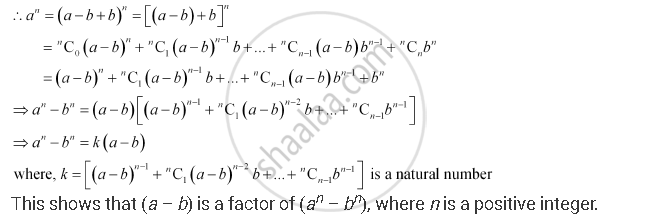CBSE (Arts) Class 11CBSE
Share
Notifications

View all notifications
Books Shortlist
Your shortlist is empty

# If A And B Are Distinct Integers, Prove That A – B Is a Factor Of An – Bn, Whenever N Is a Positive Integer. - CBSE (Arts) Class 11 - Mathematics

Login
Create free account

Forgot password?
ConceptBinomial Theorem for Positive Integral Indices

#### Question

If a and b are distinct integers, prove that a – b is a factor of an – bn, whenever n is a positive integer.

[Hint: write an = (a – b b)n and expand]

#### Solution

In order to prove that (a – b) is a factor of (an – bn), it has to be proved that

an – bn = k (a – b), where k is some natural number

It can be written that, a = a – b + bIs there an error in this question or solution?

#### APPEARS IN

Solution If A And B Are Distinct Integers, Prove That A – B Is a Factor Of An – Bn, Whenever N Is a Positive Integer. Concept: Binomial Theorem for Positive Integral Indices.
S